# Design in petroleum well drilling: length of the bottom hole assembly (BHA) required for a desired weight on bit

in StemSocial5 months ago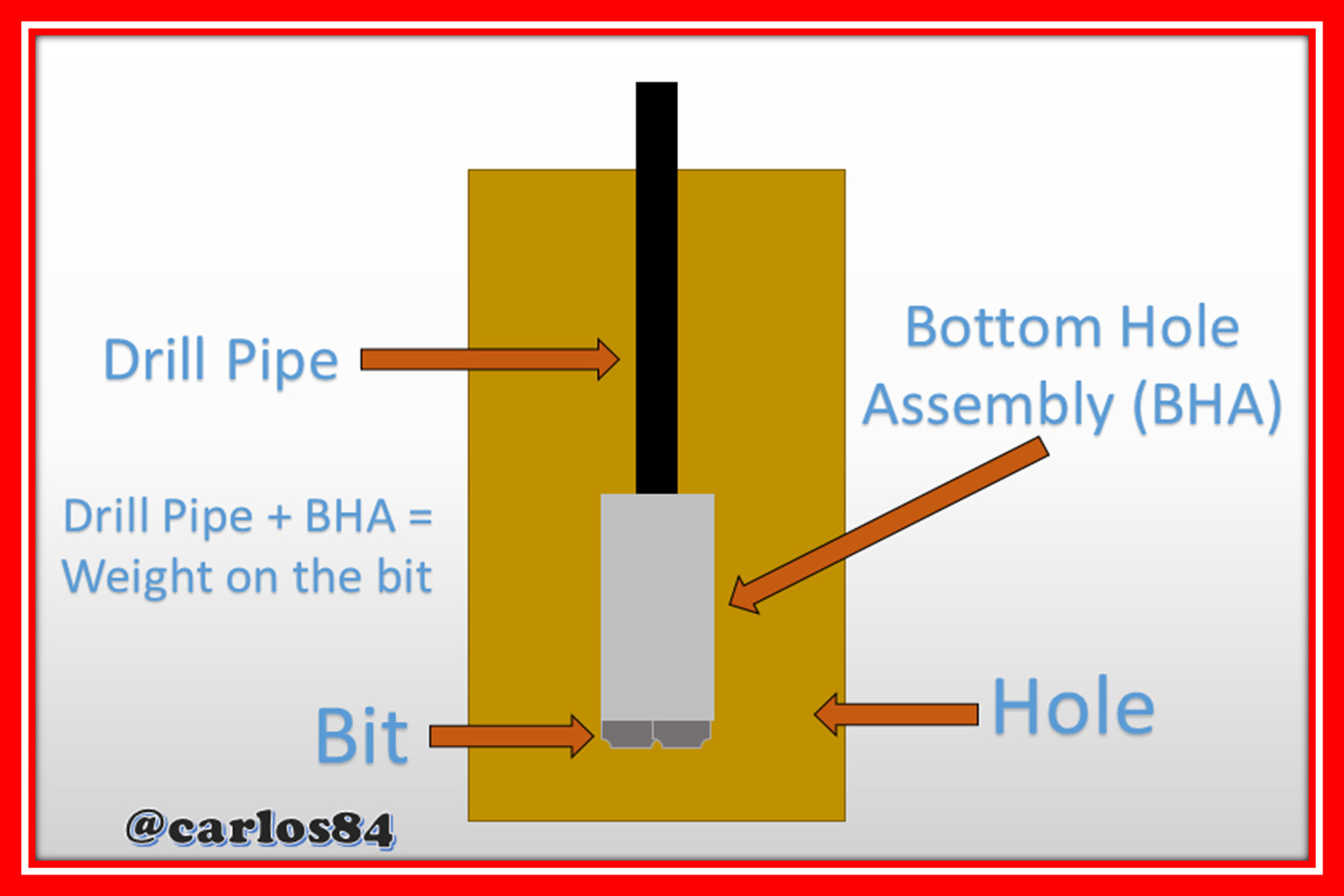Hello friends and STEM content lovers.

This time I want to share with all of you a topic that within petroleum engineering would be in the order of the design of drilling wells as is the case of calculating the length of bottom assembly required to obtain a weight on the desired or required bit depending on the design of the well drilling.

As the length of the BHA is greater, logically a greater weight on the bit will be obtained, however the requirements of the weight on the bit will be dictated by the design that is already pre-established in the drilling plan, that is why the objective of this post is to explain an exercise where an example is shown where having as data the weight on the bit (WOB), the safety factor to place the neutral point in drill collars (f), weight of the drill collar (Wdc) and finally the buoyancy factor (BF).

### Example in the calculation of the length of the bottom set required for a desired weight on the bit

The desired weight on bit (WOB) in an oil well borehole is 45000 lbs with a safety factor of 15%, having a drilling fluid density of 11 lbs/gallon, the drill collar weight is 147 lbs/ft.

To calculate the measured length in feet that can be BHA to have a weight on the wick of 45000 pounds is calculated with the following equation: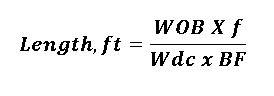In this equation we substitute in the numerator of the fraction, the multiplication of the weight on the desired bit by the safety factor, in the denominator the multiplication of the weight of the drill collar by the buoyancy factor, however we do not have the buoyancy factor calculated, but it can be calculated with the following equation: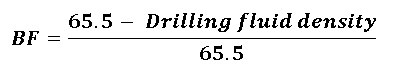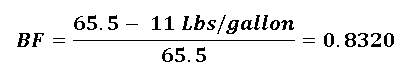Once the buoyancy factor is calculated, we calculate the measured length in feet of the bottom hole assembly (BHA) as follows: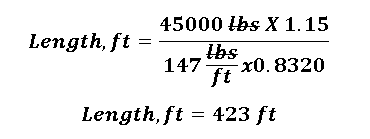### Conclusion

With 423 feet of downhole assembly tubing, a required weight on bit of 45,000 pounds is achieved, taking into consideration a safety factor of 15% and a drill collar weight of 147 lb/ft, considering the use of a drilling fluid of 11 lb/gallon density.

It is important the design that involves the necessary length of assembly at the bottom of the hole, since according to the requirements of the well, not only we estimate it due to the weight on the bit, but it is also essential to obtain a drill string rigid enough to withstand the stresses to which I have been subjected at the bottom of the hole and also flexible enough to split the sliding in the zones of the well where it is being drilled in a directional manner.

### References

Drilling BHA Components and Design

Bottom hole assembly (BHA) design for directional control